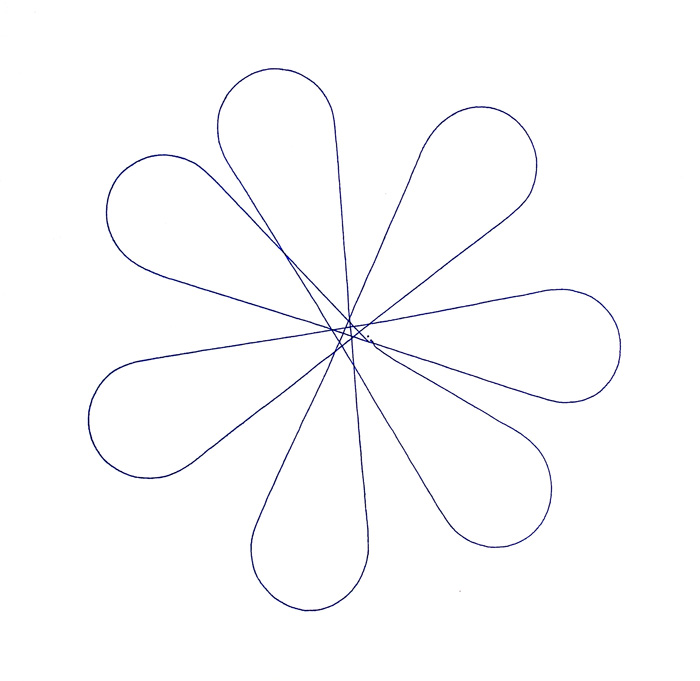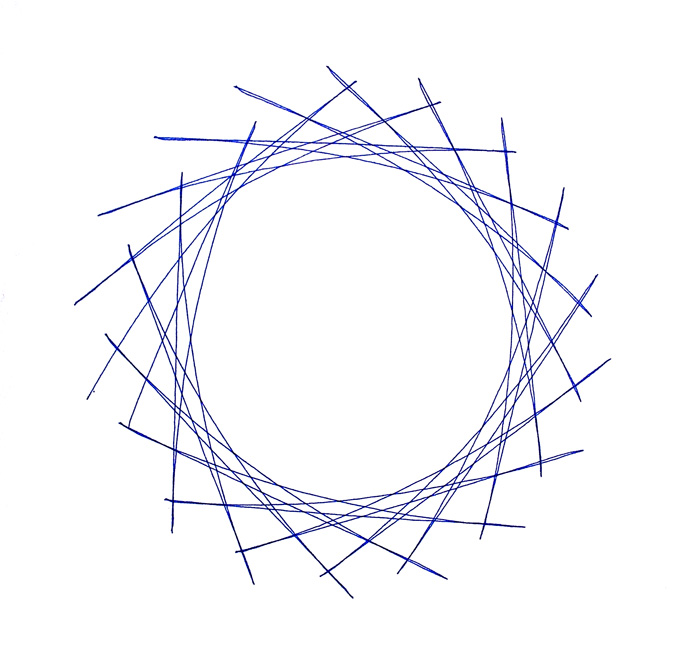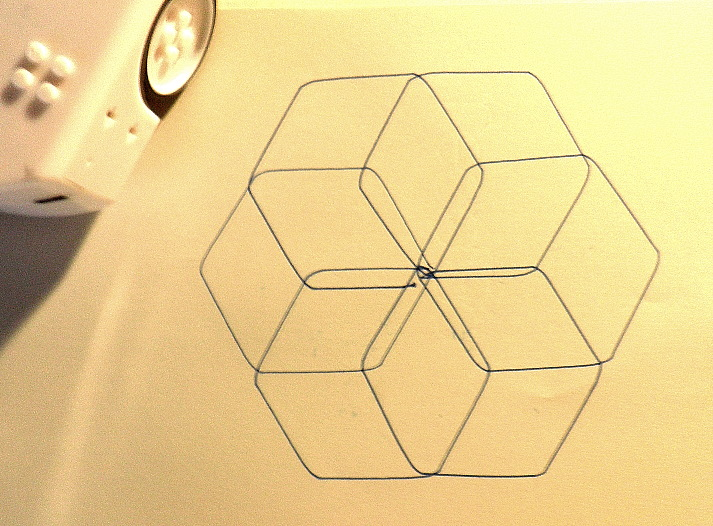# Activity:

Turn Thymio into a drawing machine by placing a thin marker in the pen holder!

# How to:

1. Copy and paste the code used in the video into Aseba Studio:

Drawing #1

``````var itera = 0

onevent temperature #Thymio updates temperature value at once per second.  You can replace this line with the following two to change time intervals
#timer.period = 1000
#onevent timer0
itera = itera + 1
if itera==1 then
motor.left.target = 230
motor.right.target = -120
end
if itera==4 then
motor.left.target = 80
motor.right.target = 80
end
if itera==7 then
itera = 0
end``````Drawing #2

``````var itera = 0

onevent temperature
itera = itera + 1
if itera==1 then
motor.left.target = 100
motor.right.target = 90
end
if itera==4 then
motor.left.target = -100
motor.right.target = -70
end
if itera==7 then
itera = 0
end``````Drawing #3

This program will have Thymio draw six hexagons around a center — to make a sort of flower.   See Tom’s Full Project here: https://www.techykids.com/hexagon-flower-2/

``````
#* SIX PETAL HEXAGON FLOWER

This program will draw six overlapping hexagons
around a center. Each part of the program will be
explained in green letters - just like these! *#

# The very first thing (ALWAYS) is to set up your variables.

var count = 0
var sides = 0     # Counts how many sides have been drawn.
var petals = 0    # Counts how many petals have been drawn.

timer.period = 2000     # A timer 'click' will happen every 2 seconds.

onevent timer0
count = count + 1    # Keeps track of how many timer 'clicks.'

#* Don't be afraid to use SUBROUTINES. Computers love them because when
they have to do the same thing over and over, calling a subroutine
is the easiest way - so YOU don't have to write the same code over and over.
In this program, all SUBROUTINES appear at the bottom.
They will be called all along the way as the program needs them. *#

#* It takes 2 actions to draw one side: drawing the line and turning.
So, it will take twelve 'counts' to make each complete hexagon.

Here we call a subroutine to draw one side of a hexagon. *#
if count == 1 or count == 3 or count == 5
or count == 7 or count == 9 or count == 11 then
callsub draw_side
end

#* After each side is drawn, a different subroutine turns Thymio clockwise.
Here we call a subroutine to turn Thymio 60 degrees to the right. *#

if count == 2 or count == 4 or count == 6
or count == 8 or count == 10 or count == 12 then
callsub turn
end

#* It takes six combination events [a side + a turn] (a total of 12) to make one "petal," so after finishing each petal (when the count reaches 13) it resets its counter to 0 and starts counting again. *#

if count == 13 then
call leds.top(255, 0, 255)    # The top turns purple when each petal is done.
motor.left.target = 73
motor.right.target = -72
petals = petals + 1    # Counting petals. 6 petals = DONE!
count = 0    # Reset counter.
end

if petals >= 6 then      # STOP altogether after drawing 6 petals
call leds.top(255,255,255)     # When the top turns white, you're done.
motor.left.target = 0
motor.right.target = 0
end

#* The SUBROUTINES -----------------------------------------------------

#*DRAW one line each time this subroutine is called.
Top color is Green *#
sub draw_side
call leds.top(0, 255, 0)
motor.left.target = 60
motor.right.target = 60
return

#* TURN 60 degrees to the right each time this subroutine is called
Top color is Red *#
sub turn
call leds.top(255, 0, 0)
motor.left.target = 73
motor.right.target = -72
return
``````